# STAT-18: Statistical Techniques for Normality Testing and Transformations

This is part of a series of articles covering the procedures in the book Statistical Procedures for the Medical Device Industry.

### Purpose

To provide guidance on normality testing to ensure the assumption of normality is adequately met when using variables sampling plans and related procedures. Related procedures include normal tolerance intervals, variables confidence limits for the proportion, and confidence statements for Ppk.  Included are procedures to handle situations when the normality test fails and procedures for the detection and handling of outliers.

### Appendices

1. General Normality Tests
2. Effects of Ties on General Normality Tests
3. Skewness–Kurtosis Specific Normality Test
4. Transforming Data
5. Sublotting Data using ANOVA
6. Sublotting Data using Kruskal–Wallis Test
7. Investigating Outliers
8. Invalidating an Outlier Value by Repeated Retesting
9. High Capability Acceptance Criteria

### Highlights

Banding Pattern Due to Ties:  If you see a normal probability plot that looks like that below, the bands are caused by the same value being repeated multiple times (ties).  When this happens both the Anderson-Darling and Shapiro-Wilk test will falsely reject normal data (p-value = 0.016).  The histogram of the data certainly looks normal.  Appendix B describes this problem.  The solution is to use the SK All (D’Agostino–Pearson) test, which is robust to ties in the data.  The SK All test is described in Appendix A and available in the validated spreadsheet STAT-18 – Skewness-Kurtosis Normality Tests accompanying the book.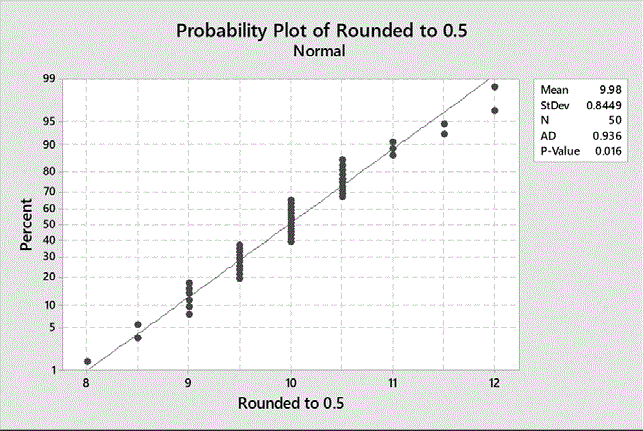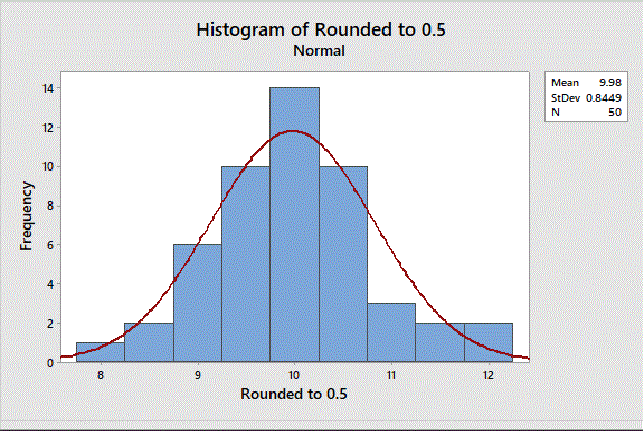• Bounded by the Normal Distribution:  Not all departures from normality invalidate the use of a variables sampling plan.  The data below fails the general normality tests due to short tails (Anderson-Darling p-value 0.0001).  The data has good capability and seems to be bounded by the normal distribution.  The only thing keeping it from passing is the failed normality test.  The SK Specific test has been designed for this purpose.  It asks the question “Can a variables sampling plan be used?” rather than “Is the data normal?”  It accepts certain departures from normality that do not invalidate the confidence statement associated with the variables sampling plan.  The SK Specific test passes this data allowing the study to pass  The SK Specific test is described in Appendix C and available in the validated spreadsheet STAT-18 – Skewness-Kurtosis Normality Tests accompanying the book.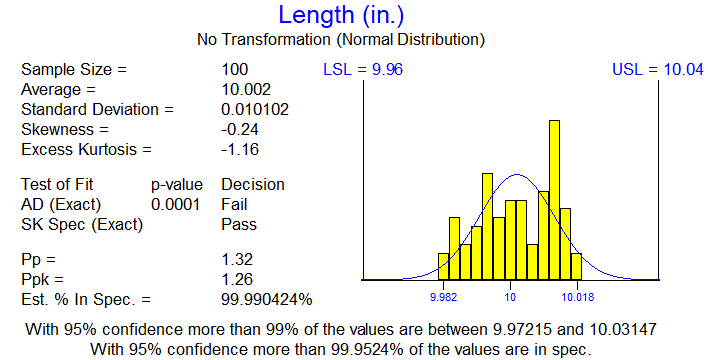• High Capability Data:  When data has high capability, a normality test may not be required.  Suppose the desired confidence statement is 95%/99% and the plan n=50, Ppk=0.96 was selected.  The data below fails the normality test.  However, since the estimated Ppk is more than 1.84 times the acceptance criteria of 0.96 and the skewness is greater than -2, the high capability acceptance criteria are meet and no normality test is required.  The high capability acceptance criteria are described in Appendix I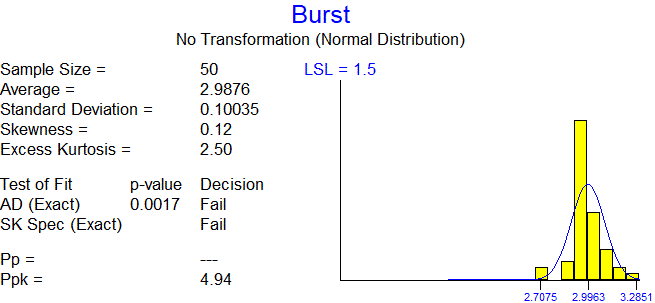• Flowchart:  The procedure provides numerous options for handling nonormal data including transforming (Appendix D), sublotting (Appendices E, F), and invalidating outliers (Appendices G, H).  It provides a step-by-step flowchart for deciding which approach to use when.  The flowchart and instructions are important in avoiding the abuse of the these methods to prevent “analysis until it passes”.

### 22 thoughts on “STAT-18: Statistical Techniques for Normality Testing and Transformations”

1.Hello, regarding the high capability data. I was reviewing your book in section STAT-18, Appendix I about this topic. The multiplier table provides multipliers for different values of reliability and sample size.
1) My first question is can I use this to justify not doing normality test before conducting normal tolerance interval analysis? (where PpK = K-factor/3)
2) Secondly, does it matter what confidence I will be testing to in my normal tolerance interval analysis? The examples your provide are for 95% confidence and 99% reliability. Can I also use this table if I plan on doing normal tolerance interval testing on 99% confidence and 99% reliability to justify not having to do normality test?

Thank you.

1.The high capability acceptance criteria also apply to Normal Tolerance Intervals. Since k=3 Ppk, apply the multiplier to the k-value. The table is specific 95% confidence. The multipliers would be somewhat higher for 99% confidence and lower for 90% confidence. The table gives multipliers for different conformance levels and sample sizes. Interpolation between table values is permitted.

2.Hello Wayne,
would it be reasonable to apply the high capability acceptance criteria on data from an unstable process? E.g. expressed by major shifts in mean and variation on samples from multiple batches?

Thank you.

1.Applying variables sampling plans when there is sizeable between lot variation is covered in Appendix C of STAT-03, Statistical Techniques for Process Validation, of my book Statistical Procedures for the Medical Device Industry. When the Between Lot standard deviation is less than 30% of the Total standard deviation, the lots are sufficiently similar that the data can be pooled with no modifications to the procedure. This includes the high capability acceptance criteria. This can be determined using a Variance Components Analysis. All points inside the control chart limits on a control chart is also sufficient evidence.

When between lot variation is greater than 30%, the effective sample size is reduced. For example, suppose there are 3 lots with 10 samples each. Then the total sample size is 30. Depending on the between lot variation, the effective sample size can vary between 30 and 3. Suppose the 10 samples from each lot are identical, meaning there is zero within lot variation but that each lot has a different value. The only variation is between lot variation. In this case, the effective sample size is 3. When there is sizeable between lot variation, the analysis and resulting confidence statements should be based on the effective sample size. It may be necessary to increase the number of lots and the number of samples per lot.

3.Hi – I was just wondering what is the statistical background/ rationale for why the “High Capability Data” is an acceptable approach?

Thank you!

1.The high capability acceptance criteria are explained on pages 584-586 on my book of Statistical Procedures. There it is demonstrated that lack of normality can greatly affect the confidence statement associated with a variables sampling plan. The high capability acceptance criteria are based on Chebyshev’s inequality. This inequality states there are limits to the number of units that can be more than 3, 4, 5 … standard deviations from the mean, regardless of the distribution. This means that if Ppk is high enough, the desired confidence statement can be made regardless of the distribution, so passing a normality test is no longer required. The problem with applying Chebyshev’s inequality is that it is based on the true mean and standard deviation and not estimates. The table of multipliers was developed through extensive simulations to determine how much the Ppk must be increased. I determined that without putting restrictions on the estimated skewness, this approach would not work in practice. I selected the restriction that the estimated skewness be between -2 and 2, because it is generally met. However, generating multipliers for other restrictions on the skewness is possible. Generating the set of multipliers provided took 5 machines 6 months to perform.

1.That seems like a novel approach to establish the multiplier and deriving the Tolerance for Non-Normal data. Is there any paper or literature that has been followed to perform this simulation? if not, are you planning to publish the method in any journal (if not already in the process of publication)?

1.Chebychev’s inequality is often used as justification for why, regardless of the distribution, there are limits on the number of units X standard deviations from the mean. For 6 standard deviations no more than 2.7778% can be out of spec. The problem with using Chebychev’s inequality is that it requires the true mean and standard deviation. The high capability acceptance criteria in my book Statistical Procedures for the Medical Device Industry expands this concept to estimates of the mean and standard deviation.

On page 584 it displays the graphic below showing the effect of the lack of normality on the protection provided by the 95%/99% n = 30, Ppk = 1.03 plan. At the RQL of 1%, there is a 5% chance of acceptance for the normal distribution (skewness = 0, kurtosis = 0). This corresponds to 95% confidence. By the time the skewness increases
to 1 or the kurtosis increases to 2, the probability of acceptance increases to 20% corresponding to 80% confidence. For much of the chart it is above 60%.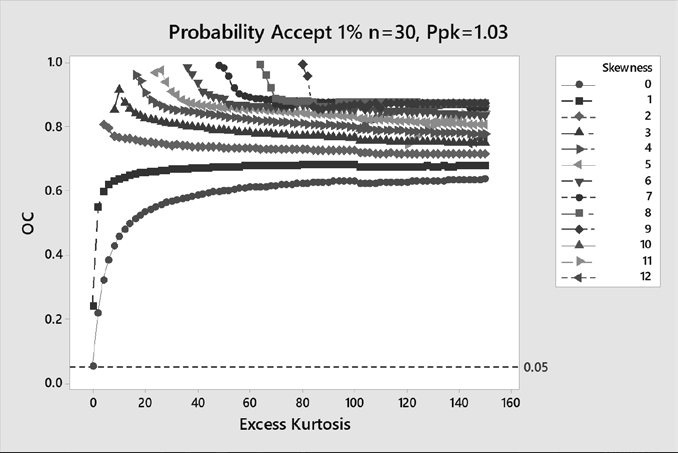This plot was generated by extensive simulation using the Johnson distribution covering all possible skewness and kurtosis values. 1,000,000 simulations were performed at each of the points.

These simulations were repeated using different values for the high capability multiplier. The figure below shows how the protection changes when a multiplier of 2.00 is applied. While there are a small number of extreme cases where the probability of acceptance is as high as 20%, the average and median probability of acceptance are below 5%.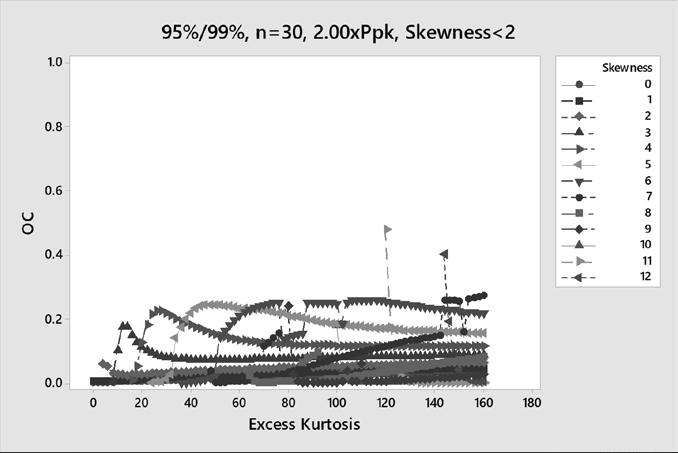4.Hello, I have a sample group for a new process which does not meet normality nor any of the non-normal distribution, in addition process does not meet stability assumption. n=60. Nevertheless, I when ahead an perform the calculations, getting a Pp = 2.05 and Ppk=1.60. My acceptance criteria is Pp>0.77 and Ppk>0.67. What would you recommend in this case? Thanks in advanced

1.Thank you, I have another question: From what I can inferred Ppk criterion is obtained from k/3, can you described how Pp criterion is calculated? Thanks in advanced.

1.In Sampling Plan Analyzer variables sampling plans with two-sided specifications have parameters k and MSD. This is the form found in ANSI Z1.4. The acceptance criteria are the sampling plan passes if Ave – k SD ≥ LSL, Ave + K SD ≤ USL, and 100 SD / (USL-LSL) ≤ MSD. MSD stands for maximum standard deviation expressed as a percentage of the specification range.

The acceptance criteria can be converted to the Ppk, Pp form using the formulas Ppk = k/3, and Pp = 100 / (6 MSD).

If one is using two-sided normal tolerance intervals rather than two-sided variables sampling plans, there is not MSD/Pp acceptance criterion. A normal tolerance interval converts to Ppk acceptance criteria only with Ppk = k/3.

A two-sided variables sampling plan is slightly more efficient than a two-sided normal tolerance interval, although the improvement is generally fairly small.

5.Can the high capability acceptance criteria be used for sample sizes less than 15? If so, how would one calculate the multiplier as the tables in your book do not include the multiplier for samples sizes of less than 15. Thank you!

1.Yes it can. However, but both the Ppk acceptance criteria and the multiplier gets increasingly higher and harder to pass. The multipliers are calculated using extensive simulation. It takes several days of computer time and an hour of my time to generate each value. The table in the book took many months to generate.

6.Dear Mr. Taylor
I reviewed STAT-18 and some questions came up regarding section 7.6 (Transformation) and the Appendix D.
In the example you are transforming the data and also the specification limit, then calculating the Ppk from the transformed data. Is it correct that also the following approach would work:
1. Transforming the data
2. Calculate tolerance intervals for the transformed data (mean +/- k*s)
3. Transform tolerance bounds back into the original units by applying the inverse of the transformation equation
4. Check if mean – k*s >= LSL, mean + k*s<= USL (mean and s of the the original data)

Do you see any problem with that? I don't because it seems to be very similar to what you are doing. I see an advantage in this, because with your example, it could be that there is the problem that the specification limits cannot be transformed. The transformation function (Johnson) is only continuous in a range near the measured values. If the specification limits are further away from the data, these cannot be transformed.
Thanks so much for the support.

1.I have no problem with that. However, the tolerance interval can still be outside the bounds of the distribution, so they cannot be reverse-transformed. Distribution Analyzer does this for you. In the second to last line below is the reverse-transformed normal tolerance interval. In this case, it 1-sided lower bound.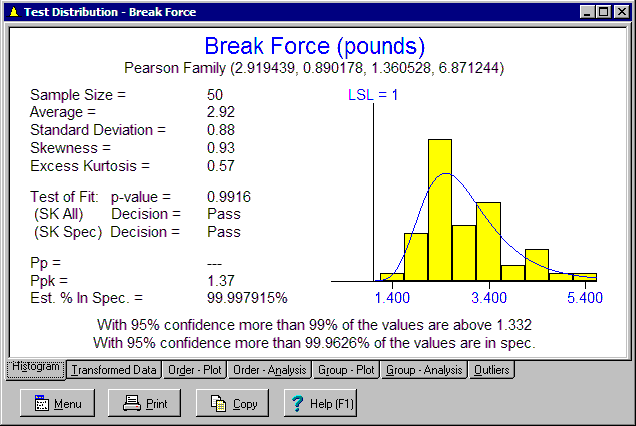Distribution Analyzer deals with the issue of bounded distributions by modifying its distribution fitting to respect specification limits. The fitted distribution’s bounds must be at least one standard deviation outside the specification limits. The bound will never be inside the specification limit where the specification limit cannot be reverse-transformed. It also prevents a bound from being barely outside the specification limits resulting in misleading results. Your tolerance interval approach would still benefit from this modification to the distribution fitting. Many companies have criticized the Johnson Transformation in Minitab because of this issue and some even ban the use of the Johnson Transformation in Minitab. However, the problem is not with the Johnson Distribution. It is with the way bounds are handled. The same problem can occur with other distributions.

7.What would be the multiplier, Pp and Ppk for the sample sizes above 100 units.
Should we use the same values as for 100?
Thanks.

1.The multipliers decrease as the sample size goes up. It would be valid, but a conservative approach, to apply the multipliers for n=100 to higher sample sizes.

8.Wayne,
Can the non-normal high capability multipliers for Ppk/Pp as defined in STAT 18 Appendix I be utilised for a performance qualification requirement n=30 Cpk >1.33.
Thanks,
JK

1.Cpk does not consider whether the process is stable or not. Half the time the process could be entirely below the lower spec and half the time entirely above the upper spec. The Cpk could be 1.33 but the product 100% nonconforming. Assuming there is an additional requirement of a stable process, then Cpk = Ppk.

For n=30, Ppk > 1.33, Sampling Plan Analyzer can be used to determine this sampling plans has an RQL(0.05)= 0.1125% nonconforming making it a 95%/99.89% plan. This is a little below 95%/99.9% so the 95%/99.9% high capability multiplier can be used. The n=30, 95%/99.9% high capability multiplier is 3.58 from page 585 of the book of procedures. Applying this to the acceptance criteria of 1.33, the high capability acceptance criteria is Ppk > 1.33 x 3.58 = 4.76. If Ppk is greater than 4.76, normality is no longer required and the 95%/99.9% statement can be made.

9.Nguyễn Nhật Lam

Hi Wayne,
I have a problem with a data set. Here are what I obtain from Minitab:
– Mean ~ median (13.046 ~ 13.245 lbf). All value > 5 lbf.
– Ppk = 3.16 > 2.06.
– Skewness = -2.11 5 lbf.
With an exceeding by 0.11 in Skewness, and the value in your book is just “-2” without any decimal places. Can I accept this data set as meeting the high capability criteria as -2.11 can be rounded up to -2 with zero decimal?

Note: SK specific test fails, transformation is ok but normal tolerance interval fails, FYI.

1.For the high capability acceptance criteria, the limits of -2 to 2 are exact values so values should not be rounded when comparing them to these limits. I have started generating tables of high capability acceptance criteria like -3 to 3. If you provide your C/R value and sample size, I can check if I have generated a value for your case.

Scroll to Top